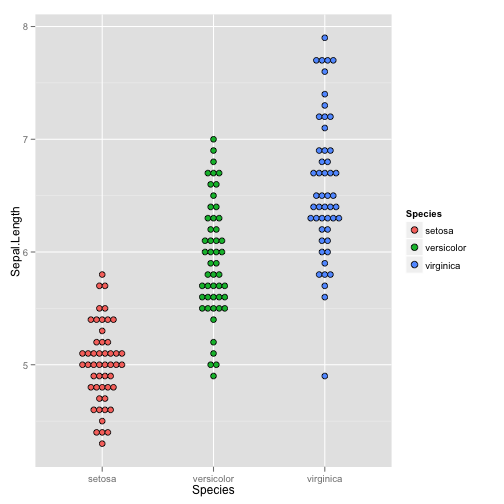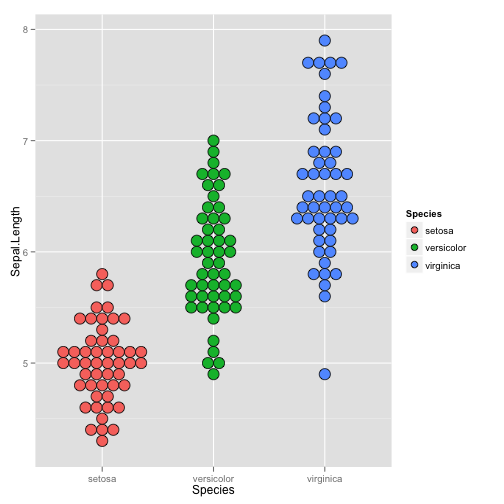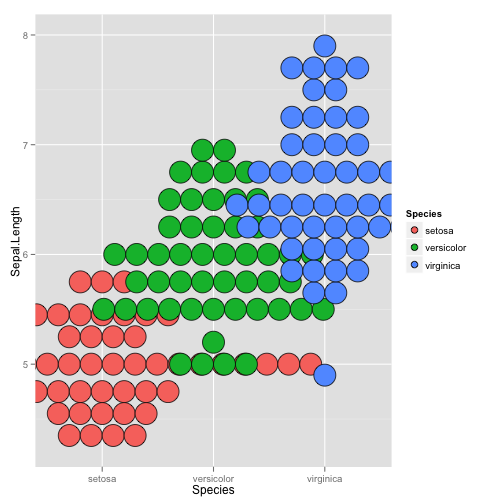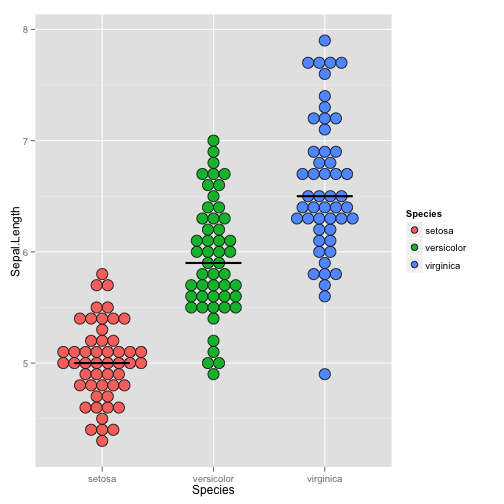# geom_dotplot() with median line

``````## Settings for RMarkdown http://yihui.name/knitr/options#chunk_options
opts_chunk\$set(comment = "", warning = FALSE, message = FALSE, echo = TRUE,
tidy = FALSE, fig.width = 7, fig.height = 7)
options(width = 116, scipen = 10)
``````

## Simple plots. See changes due to different binwidth.

``````library(ggplot2)

ggplot(data = iris) +
geom_dotplot(aes(y = Sepal.Length, x = Species, fill = Species),
binaxis = "y",         # which axis to bin along
binwidth = 0.05,       # Minimal difference considered diffeerent
stackdir = "center"    # Centered
)
````````````

ggplot(data = iris) +
geom_dotplot(aes(y = Sepal.Length, x = Species, fill = Species),
binaxis = "y",         # which axis to bin along
binwidth = 0.1,        # Minimal difference considered diffeerent
stackdir = "center"    # Centered
)
````````````

ggplot(data = iris) +
geom_dotplot(aes(y = Sepal.Length, x = Species, fill = Species),
binaxis = "y",         # which axis to bin along
binwidth = 0.2,        # Minimal difference considered diffeerent
stackdir = "center"    # Centered
)
````````````ggplot(data = iris, aes(y = Sepal.Length, x = Species)) + # Move y and x here so than they can be used in stat_*